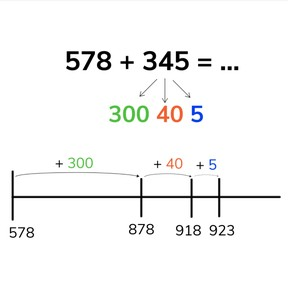No account needed.8,000 schools use Gynzy92,000 teachers use Gynzy1,600,000 students use Gynzy

## General

Students learn to add to 1,000 by splitting the second addend to make it easier to add to the first addend. These addition problems are more challenging as they involve adding to the next place value. For example: 578 + 345 = 923

2.NBT.B.7

## Relevance

Discuss with the students that it is useful if you can add by splitting the second addend. By doing so, you make adding larger numbers quicker and easier.

## Introduction

Show the addition pyramid on the interactive whiteboard. Point at an addition problem and ask a student to give the total. Erase the squares to show the answer. Ask students to read the story problem and ask them to determine the math problem and to solve for the total. Students can choose from given answers. Ask students how they determined which answer was correct.

## Development

Check that students are able to add to 1,000 using grouping by asking the following questions:
- Why is it useful to be able to add using grouping?
- What can you do to make it easier to add beyond the tens? (regrouping)

## Guided practice

Students first solve an addition problem using the number line. They must add the second addend in jumps. They are then asked to solve by grouping.

## Closing

Discuss with students why it is important to be able to add to 1,000 by splitting the second addend because it makes adding larger numbers easier. Students then work in pairs to practice adding on the number line. The first student writes a number at the start of the number line. They then determine a total and take turns jumping in hundreds, tens or ones to reach that total. The pairs are then asked to play memory. The one card has an addition problem, and the second card shows the total to that addition problem.

## Teaching tips

Students who have difficulty can make use of the number line. Ask them to draw all the jumps on the number line. Make sure that they know to first jump the hundreds, then the tens, and finally the ones.

## Instruction materials

Addition memory: make sets with addition problems to 1,000 on one card, and the total on a second card.

### The online teaching platform for interactive whiteboards and displays in schools

• Save time building lessons

• Manage the classroom more efficiently

• Increase student engagement